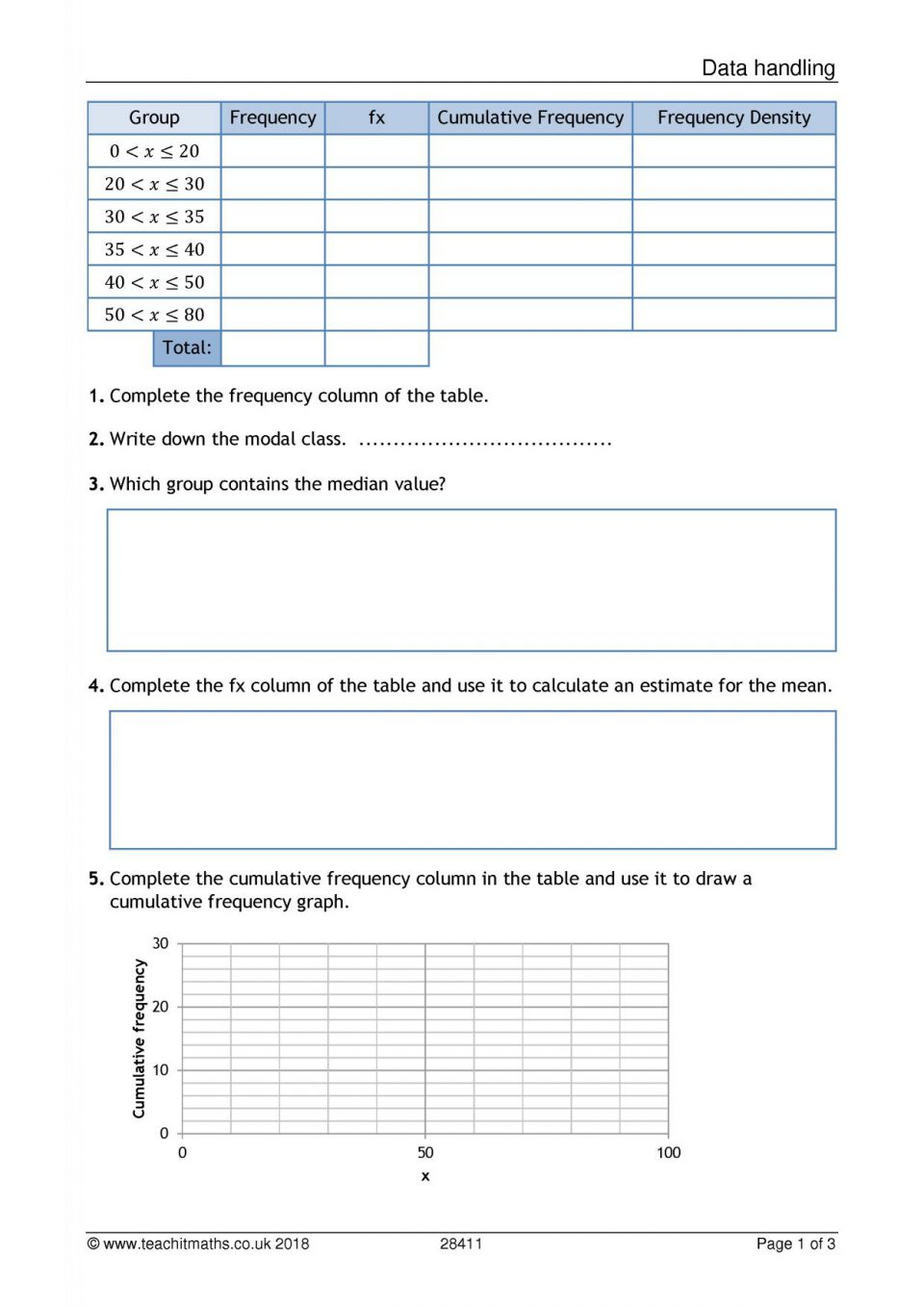Maths

# Data processing

### Simple average

Example:
During the first 10 days of the football Championship, Greg’s club won 4 matches, made 3 draws and wiped out 3 detours.
The average number of points per game of the club is calculated.
The squad is 10 (10 matches were played).

The sum of the points is 4 × 3 points (WINS) plus 3 × 1 point (dummies) plus 0 (defeats), i.e. 12 + 3 + 0 = 15 points.

The average is therefore \frac{15}{10} = 1.5 point.

Greg’s club got an average of 1.5 points per game in the beginning of the championship.

### Weighted average

Weighting by coefficients

Weighted is the adjective derived from the name weight: In a data set, one can assign a coefficient to each value. The larger the coefficient, the more weight the data has.
The weighted average is the quotient of the sum of the products of each value and its coefficient, divided by the sum of the coefficients.

Example:
Aziz’s teacher assigns factors to homework: 1 for homework, 2 for surprise checks, and 4 for homework in one hour.
Here are the notes of Aziz in the first quarter, with the coefficient of each:

 Note 12 8 15 14 10 11 Coefficient 1 2 4 1 2 4

The sum of the coefficient is 1 + 2 + 4 + 1 + 2 + 4 = 14
The sum of the weighted scores is 1 × 12 + 2 × 8 + 4 × 15 + 1 × 14 + 2 × 10 + 4 × 11 = 166